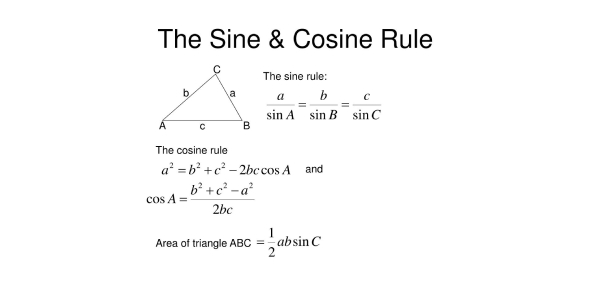# Trigonometry Trivia Quiz: Cosine And Sine Rule!

10 Questions | Total Attempts: 725Settings.

• 1.
A/sin A = b/______
• 2.
In the above diagram, AB = 100cm, BC = 80cm and ABC = 130 degreesWhat is the difference between the length of AB + BC and AC?Leave your answer to one decimal placeRemember to put "cm"
• 3.
From the previous question, find the shortest length from B to ACHint: Start by finding the area of the triangle ABCleave your answer to one decimal placeremember to put "cm^2"
• 4.
In the diagram above, angle WZY is 60 degrees, angle XWY is 120 degrees, WX = 7m and WY = 8m.Find the length of YZRemember to put "m"
• 5.
From the previous question, find the length of XYRemember to put "m"
• 6.
From the previous question,find the shortest length from Z to WYHint: start by finding the area of the triangleLeave your answer to three significant figuresRemember to put "m^2"
• 7.
In the diagram above, AD = 2.8m, BE = 4.1m, angle ADC is a right angle, angle DAE = 74 degrees, angle AEB = 123 degrees and AEC is a straight lineFind ACLeave your answers to one decimal placeRemember to put "m"
• 8.
From the previous question, find ABLeave your answers to three significant figuresRemember to put "m"
• 9.
From the previous question,if F is a point on AE such that DF is parallel to BE, find AFLeave your answers in three significant figuresRemember to put "m"
• 10.
From the previous question,calculate the area of the triangle ADFLeave your answer to one decimal placeRemember to put "m^2"
Related TopicsBack to top